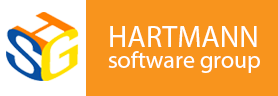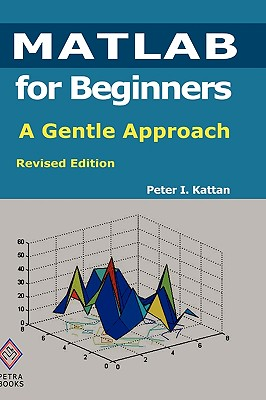### MATLAB for Beginners: A Gentle Approach: Revised Edition### Huge savings for students

Each student receives a 50% discount off of most books in the HSG Book Store. During class, please ask the instructor about purchase details.
 List Price: \$57.49 Price: \$28.74 You Save: \$28.74
0This book is written for beginners and students who wish to learn MATLAB. One of the objectives of writing this book is to introduce MATLAB to students in high schools. The material presented is very easy and simple to understand - written in a gentle manner. The topics covered in the book include arithmetic operations, variables, mathematical functions, complex numbers, vectors, matrices, programming, graphs, solving equations, and an introduction to calculus. In addition, the MATLAB Symbolic Math Toolbox is emphasized in this book. There are also over 230 exercises at the ends of chapters for students to practice. Detailed solutions to all the exercises are provided in the second half of the book. The author has been using MATLAB for the past fifteen years and is the author of the best selling book "MATLAB Guide to Finite Elements". For the paperback edition, visit Amazon.com. This description applies to the Revised Edition.
Peter I. Kattan